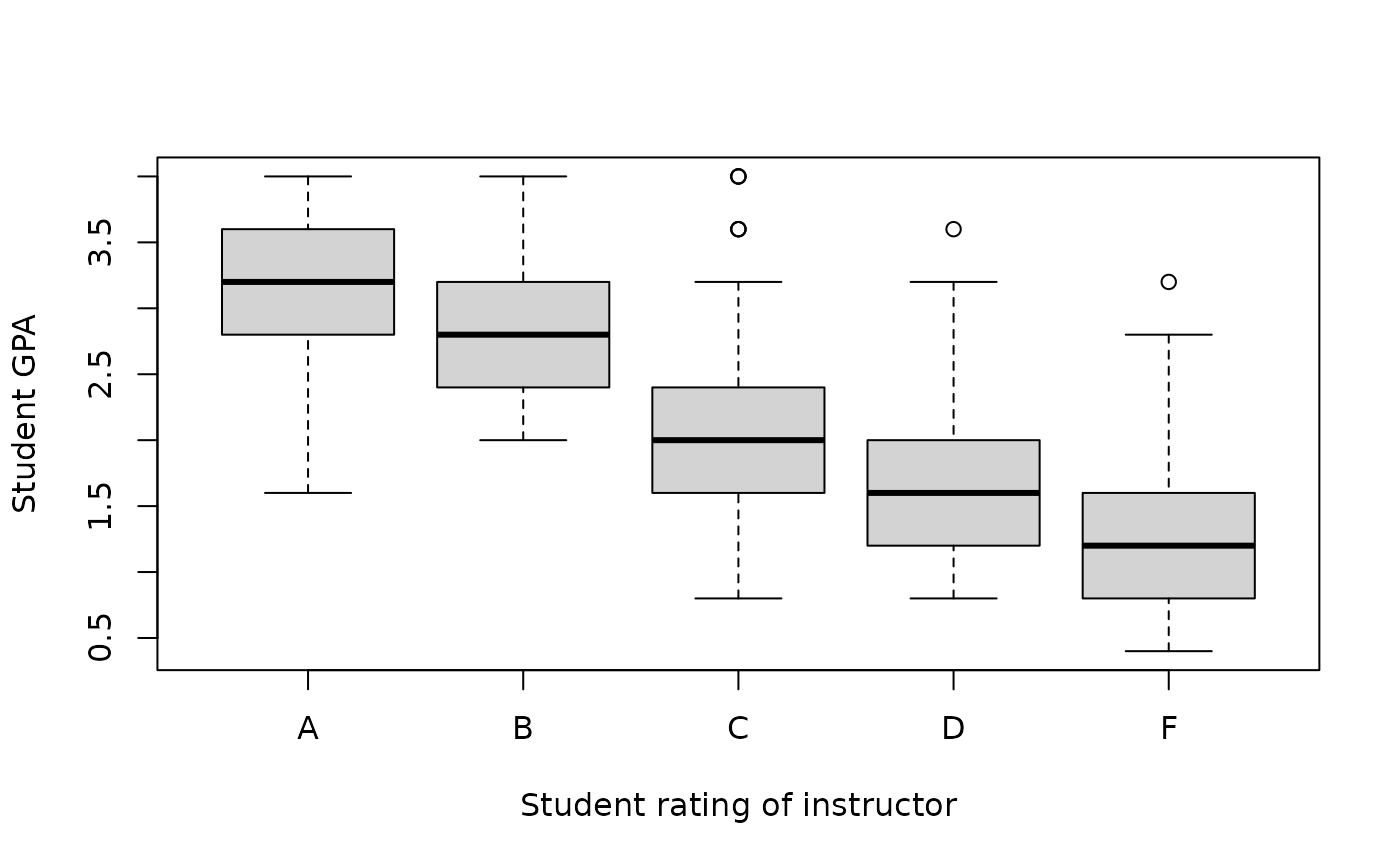Data for Example 2.6

Ratings

## Format

A data frame/tibble with 250 observations on two variables

rating

character variable with students' ratings of instructor (A-F)

gpa

## Examples


boxplot(gpa ~ rating, data = Ratings, xlab = "Student rating of instructor",
ylab = "Student GPA")if (FALSE) {
library(ggplot2)
ggplot2::ggplot(data = Ratings, aes(x = rating, y = gpa, fill = rating)) +
geom_boxplot() +
theme_bw() +
theme(legend.position = "none") +
labs(x = "Student rating of instructor", y = "Student GPA")
}